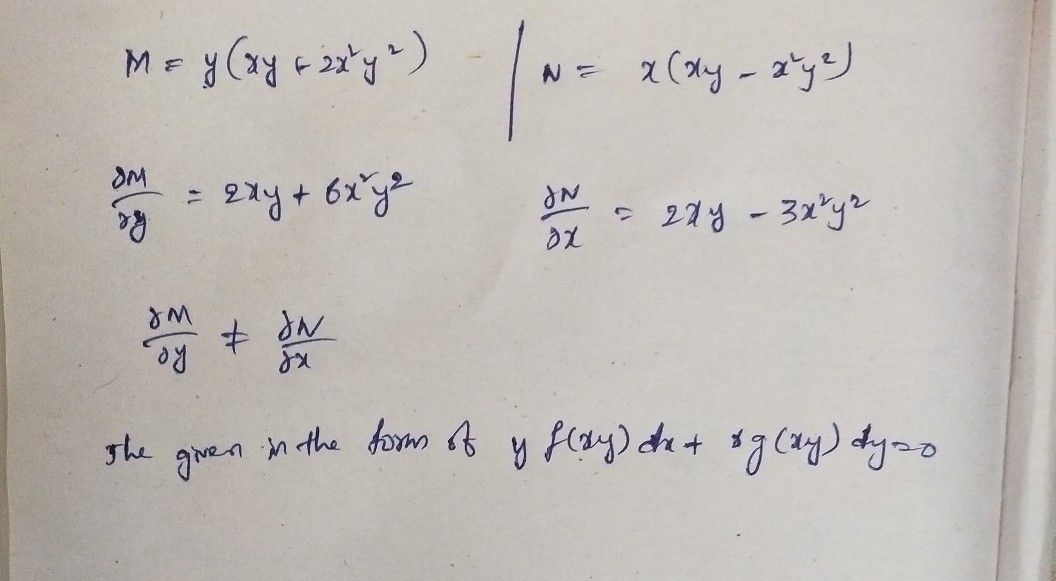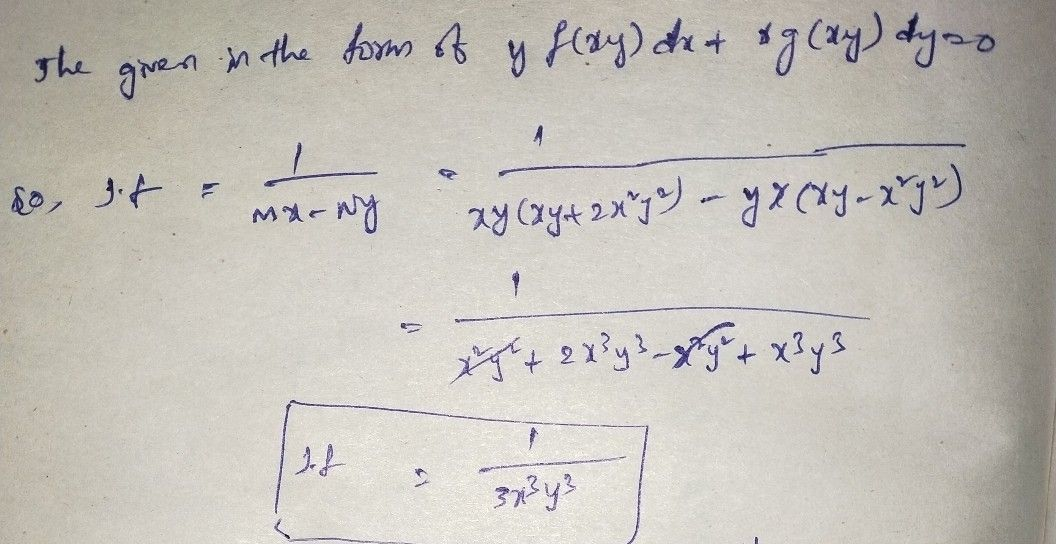Symbol
Problem$The$ integrating factor of $y\left(xy+2x^{2}y^{2}\right)dx+x\left(xy-x^{2}y^{2}\right)dy=0$ is $1\right)$ $\dfrac {1} {3x^{3}y^{3}}$ $2\right)$ $\dfrac {1} {x^{3}}$ $3\right)$ $\dfrac {1} {y^{3}}$ $4\right)$ $-\dfrac {1} {3x^{3}y^{3}}$
10th-13th grade
Calculus
Search count: 106
SolutionQanda teacher - sweetie